# Energy Stored on a Capacitor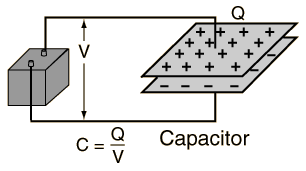The energy stored on a capacitor can be calculated from the equivalent expressions: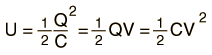This energy is stored in the electric field.

 A capacitor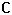=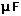= x 10^ F
which is charged to voltage V= V
will have charge Q = x10^ C
and will have stored energy E = x10^ J.

From the definition of voltage as the energy per unit charge, one might expect that the energy stored on this ideal capacitor would be just QV. That is, all the work done on the charge in moving it from one plate to the other would appear as energy stored. But in fact, the expression above shows that just half of that work appears as energy stored in the capacitor. For a finite resistance, one can show that half of the energy supplied by the battery for the charging of the capacitor is dissipated as heat in the resistor, regardless of the size of the resistor.

 Derive expressions
Index

Capacitor Concepts

 HyperPhysics***** Electricity and Magnetism R Nave
Go Back

# Electric Field Energy in Capacitor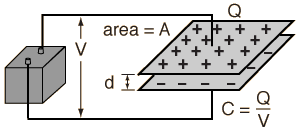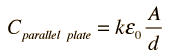Show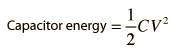Show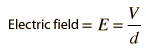Show

The energy stored on a capacitor is in the form of energy density in an electric field is given by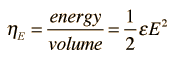This can be shown to be consistent with the energy stored in a charged parallel plate capacitor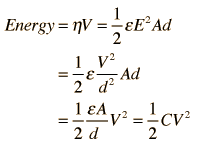Energy stored in capacitor
Index

Capacitor Concepts

 HyperPhysics***** Electricity and Magnetism R Nave
Go Back